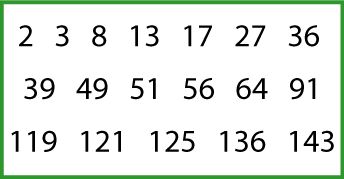#### You may also like### Consecutive Numbers

An investigation involving adding and subtracting sets of consecutive numbers. Lots to find out, lots to explore.### Tea Cups

Place the 16 different combinations of cup/saucer in this 4 by 4 arrangement so that no row or column contains more than one cup or saucer of the same colour.### Chocolate

There are three tables in a room with blocks of chocolate on each. Where would be the best place for each child in the class to sit if they came in one at a time?

# Number Families

## Sets of Numbers

How many different sets of numbers with at least four members can you find in the numbers in this box?For example, one set could be multiples of $4$ {$8, 36 ...$}, another could be odd numbers {$3, 13 ...$}.

### Why do this problem?

This problem gives plenty of opportunity for both discussion and exploring patterns, properties and relationships involving numbers. It also helps learners to make general statements about properties of numbers such as squares, factors, multiples, odd and even, and to identify examples for which a statement is true or false.

### Possible approach

You could also use this interactivity as a starter to this problem where you drag numbers you "like" (i.e. are part of a set) to one side and numbers you "don't like" (i.e. are not in your set) to the other. The children then have to ask questions with yes/no answers to determine the name of your set. You could invite some learners to take the lead on this themselves, with the rest of the group asking questions.

You could then introduce the problem itself and learners could work in pairs from this sheet so that they are able to talk through their ideas with a partner. (The sheet has two copies of the box of numbers on it.) You could also challenge pairs to find two other possible members for their sets from the numbers under $150$.

At the end learners could be asked for the titles of the sets they have found and then others could suggest members for these sets. You could make statements such as "$9$ is a member of this set" and ask learners to identify whether the statement is true or false. When sets of multiples of $2$, $4$ and $8$ have been found and discussed, learners could be asked to explain why no other sets of multiples of even numbers are possible from the numbers in the box.